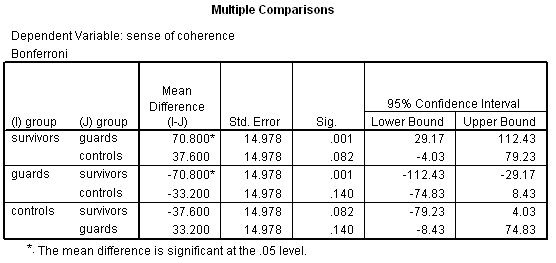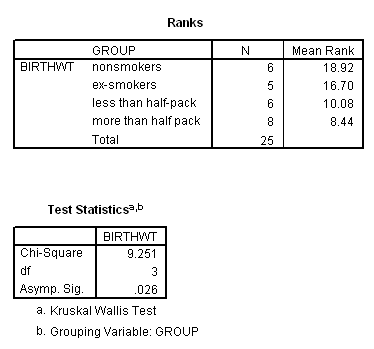# Review Questions

1. False.
2. (a) descriptive statistics (b) side-by-side graphs (c) ANOVA (d) Kruskal-Wallis
3. Because you have concluded there are no significant differences among means.
4. When k = 3, there are 3 post hoc comparisons (1 v. 2, 1 v. 3, and 2 v. 3). When k = 4, there are 6 post hoc comparisons (1 v. 2, 1 v. 3, and 1 v. 4, 2 v. 3, 2 v. 4, 3 v. 4). In general, there will be kC2 = k! / [(n)!(n-k!)] comparisons. For example, when k = 5, there are 5C2 = 5! / [(3)!(2!)]  = 5À4À3! / [(3!)(2À1)] = 20 / 2 = 10.
5. Because the variance estimate is based on pooling the data from all k groups.
6. No selection bias; No information bias; No confounding.
7. Independence, Normality, Equal variances.
8. Validity assumptions are of greater importance than distributional assumptions because "bias trumps variability." Many statistical tests are robust against violations of distributional assumptions but are not robust against violations in validity assumptions. In addition, invalid data corrupts descriptive and exploratory methods, not just inferential methods.
9. (1) EDA (2) Levene's test
10. H0: the variances in the k populations are equal versus  H1: at least one population variance differs
11. Because it is more robust (does not need evidence of Normality or unequal variance).
12. H0: the k populations have equal locations H1: at least one populations differs
13. (1) Descriptively (2) K-W test (3) Transform the data to a different scale that makes the variances more equal and then do ANOVA
14. Vocabulary words -- see lecture notes for insights

13.1 Weight gain and junk food. -- This exercise is similar to Lab 3 in HS 267

(A) Post hoc -- LSD method
H0: Á1 = Á2; tstat = (13.14- 3.44) / 0.5586 = -4.12, df = 12; P = 0.0014; highly significant (reject H0)
H0: Á1 = Á3; tstat = (13.14-9.14) / 0.5586 = 3.58, df = 12; P = 0.0038; highly significant
H
0: Á2 = Á3; tstat = (13.44 - 9.14) / 0.5586 = 7.69, df = 12; P @ .0000; highly significant
Conclusion: all three groups differ significantly. We can be confident in rejecting all three the null hypotheses.

(B) Post hoc -- Bonferroni's method
H0: Á1 = Á2; P = 0.0014 Î 3 = .0042; highly significant ( reject H0)
H0: Á1 = Á3; P = 0.0038 Î 3 = .0114; significant
H0: Á2 = Á3; P @ .0000 Î 3 = .0000; highly significant

(C) SPSS computations should be identical to yours, except for rounding error.

(D) List the three types of systematic errors ( biases) that may effect statistical analyses.  (1) Information bias (2) Selection bias (3) Confounding.

(E) In addition to the validity assumptions, ANOVA has distributional and sampling assumptions. Name these. (1) Sampling independence (2) Normality of sampling error (3) Equal variance

(F) Test the groups for unequal variance using Levene's procedure via SPSS. H0: s21 = s22 = s23 vs. H1: at least one population variance differs, FLevene = 2.45 with 2 and 12 df; P = 0.13 .The difference in variances is not significant.

(G) Conduct a Kruskal-Wallis test with SPSS.  H0: population have equal averages vs. H1: at least one population average differs. c2K-W = 11.18, df = 2, P = 0.004 (via SPSS) The groups differ significantly.

13.3 The problem of multiple comparisons.

(A) What is the probability of making 3 correct independent decisions in a row? 0.993 = 0.9703 What is the probability of getting at least 1 of the three wrong? 1 - 0.9703 = 0.0297

(B) What is the probability of making 10 correct independent decisions in a row? 0.9910 = 0.9044  What is the probability of getting at least 1 wrong out of the 10? 1 - 0.9044 = 0.0956
(C) What is the probability of making 20 correct independent decisions in a row? 0.9920 = 0.8179 What is the probability of making at least one incorrect decisions out of the 20? 1 - 0.1821 = 0.1821

13.5 Sense of coherence. Conduct post-hoc comparisons using a Bonferroni adjustment. Summarize the results. The SPSS output below shows a significant difference between survivors and guards (P = 0.001), and a marginally significant difference between survivors and controls (P = 0.082).13.7 Maternal adaptation (Howell, 1995, pp. 302 - 304; mat-role.sav). Place holder.

13.8 - 13.20 reserved for future use

13.21 Testosterone levels in roosters (visual inspection of boxplots). ... would you perform ANOVA on these data? Explain your reasoning. No. Variances differ by wide margins.

13.23 Smoking and birth weight, unequal variances? Rebut this criticism .... The sample standard deviations do not vary much, ranging from 1.17 to 0.72. No standard deviations is more than twice any another. (You can tell a lot about variability by looking at sample standard deviations.)

13.25 Smoking and birth weight (K-W).  Conduct a K-W procedure, and compare its results to those achieved by one-way ANOVA. Output (below) reveals X2stat = 9.25 with 3 df, P = 0.026. This P value is nearly the same as the one produced by ANOVA.